# Density - simple example

Material of density of 532 kg/m3 occupies a container volume of 79 cm3. What is its mass?

Correct result:

m =  42 g

#### Solution: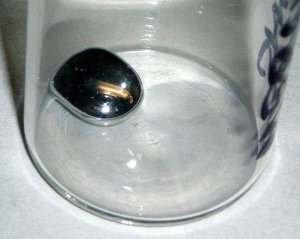We would be pleased if you find an error in the word problem, spelling mistakes, or inaccuracies and send it to us. Thank you!Tips to related online calculators
Check out our ratio calculator.
Do you know the volume and unit volume, and want to convert volume units?
Tip: Our Density units converter will help you with the conversion of density units.
Do you want to convert mass units?

## Next similar math problems:

• Here isHere is a data set (n=117) that has been sorted. 10.4 12.2 14.3 15.3 17.1 17.8 18 18.6 19.1 19.9 19.9 20.3 20.6 20.7 20.7 21.2 21.3 22 22.1 22.3 22.8 23 23 23.1 23.5 24.1 24.1 24.4 24.5 24.8 24.9 25.4 25.4 25.5 25.7 25.9 26 26.1 26.2 26.7 26.8 27.5 27.6 2
• Tower modelTower height is 300 meters, weight 8000 tons. How high is the model of the tower weight 1 kg? (State the result in the centimeters). The model is made from exactly the same material as the original no numbers need to be rounded. The result is a three-digi
• Cu thiefThe thief stole 121 meters copper wire with cross-section area of 103 mm2. Calculate how much money gets in the scrap redemption, if redeemed copper for 4.6 eur/kg? The density of copper is 8.96 t/m3.
• Engineer KažimírThe difference between politicians-demagogues and reasonable person with at least primary education beautifully illustrated by the TV show example. "Engineer" Kažimír says that during their tenure there was a large decline in the price of natural gas, pri
• BricksOpenings in perforated bricks occupy 10% and brick has dimensions 30 cm, 15 cm and 7.5 cm. Calculate a) the weight of a perforated bricks, if you know that the density of the full brick material is p = 1800 kg/m3 (1.8 kg/dm3) b) the number of perforated
• Basic formExpressed the ratios of values in the basic form: 0,5 t : 1,2 kg 200 l : 0,15 m3 12 t : 3600 kg 500 kg : 2,5 t 0,9 kg : 500 g 3,6 m : 240 cm 1200 mm : 2,4 m 300 l : 0,3 m3 6 min 30 s : 900 s
• Railway wagonThe railway wagon holds 75 m3 load. Wagon can carry a maximum weight of 30 tonnes. What is the maximum density that may have material with which we could fill this whole wagon? b) what amount of peat (density 350 kg/m3) can carry 15 wagons?
• PumpsPump that draws water at velocity 3.5 liters per second water from a construction trench take 35 minutes. a) Find out how many minutes the water would run out of the trench pump that draws 7.4 liters of water per second. b) What is the pumping velocity wo
• Used cars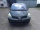Peter plans to buy a used car: the first car Renault Espace 2.0 dCi 16V Dynamique 2006, costs 2000 euros. It is 14 years old and has a combined diesel consumption of 8 liters. / 100 km. Diesel costs 1.1 euros/liter. How much will the car cost him to opera
• PumpWhat power has a pump output to move 4853 hl of water to a height of 31 m for 8 hours?
• The prison ballCalculate the density of the material that the prison ball is made from if you know its diameter is 15cm and its weight is approximately 2.3kg. With the help of mathematical-physicochemical tables estimate what material the ball is made from.
• GearsThe front gear on the bike has 32 teeth and the rear, on the wheel, has 12 teeth. How many times does the rear wheel of the bike turns if you turn the right pedal 30 times? What distance will you go if the circumference of the bicycle wheel is 250 cm?
• Coke and coal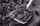20.1 tons of coke is produced from 30 tons of black coal. How much coke is made from 1 kilogram of coal?
• Cooling liquid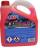Cooling liquid is diluted with water in a ratio of 3:2 (3 parts by volume of coolant with 2 volumes of water). How many volumes of coolant must be prepared for a total 0.7 dm3 (liters) of the mixture?
• Sugar 9150 kg of sugar beet yields 24 kg of sugar. From how many tons of sugar beet can be obtained three tons of sugar?
• Wood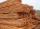Wood contains 12% water. 1 m3 of wood weighs 650 kg. How many liters of water it contains?
• Copper plateCalculate the thickness of the copper plate with a density 8.7 g/cm³ measuring 1.5 meters and 80 cm and its weight is 3.65 kg